SuSiE manuscript

# A pedagogical example of fine-mapping problem¶

Here we demonstrate with simulated data a setting of fine-mapping problem where it is clearly problematic to run forward stagewise selection, but SuSiE's Iterative Bayesian Stepwise Selection procedure can correctly identify the causal signals and compute posterior inclusion probability (posterior mean from a variational approximation to the posterior distribution). We also compared SuSiE results with other Bayesian sparse regression approach, and demonstrate that SuSiE is robust to prior choice and provide information more relevant to fine-mapping applications that other methods do not provide.

From simulated data we pick this case example where the top z-score might be a result of two other nearby signal clusters (containing variables of non-zero effects). See here for a brief description on the simulation settings.

In :
%revisions -s -n 10

Revision Author Date Message
642561b Gao Wang 2020-02-12 Assess property of an intermediate CS
ab9fad1 Gao Wang 2020-02-12 Change text on plots
baa6a01 Gao Wang 2020-02-06 Add plot for the 1st iteration
c2bc3fb Gao Wang 2020-01-20 Add LD heatmap code
5a0d38d Gao Wang 2020-01-14 Add a notebook on identifiability
0a00661 Gao Wang 2019-07-10 Change plot color
fe1be96 Gao Wang 2018-12-18 Adjust figures 1 and 5
83af7a7 Gao Wang 2018-12-16 Update benchmark instruction and documentations
e391550 Gao Wang 2018-12-16 Update notebook narratives
593cb30 Gao Wang 2018-12-14 Improve figures
In :
library(susieR)
set.seed(1)

In :
data("N2finemapping")
attach(N2finemapping)

In :
dat = N2finemapping
names(dat)

1. 'X'
2. 'chrom'
3. 'pos'
4. 'true_coef'
5. 'residual_variance'
6. 'Y'
7. 'allele_freq'
8. 'V'

This simulated data-set has two replicates. We focus on the fist replicate:

In :
r = 1


## Comparing SuSiE with per-variable regression¶

We fit SuSiE and obtain its CS and PIP. We also perform a per-feature simple regression and get (asymptotic) z-scores by setting compute_univariate_zscore to TRUE.

In :
fitted = susie(dat$X, dat$Y[,r], L=5,
estimate_residual_variance=TRUE,
scaled_prior_variance=0.2,
tol=1e-3, track_fit=TRUE,
compute_univariate_zscore=TRUE,
coverage = 0.95, min_abs_corr = 0.1)


To show intermediate state of the IBSS algorithm we run the same analysis but set max_iter to 1,

In :
fitted.one.iter = susie(dat$X, dat$Y[,r], L=5, max_iter=1,
estimate_residual_variance=TRUE,
scaled_prior_variance=0.2,
tol=1e-3,
coverage = 0.95, min_abs_corr = 0.1)

Warning message in susie(dat$X, dat$Y[, r], L = 5, max_iter = 1, estimate_residual_variance = TRUE, :
“IBSS algorithm did not converge in 1 iterations!”


We plot p-values from per-feature regression in parallel with PIP from SuSiE.

In :
b = dat$true_coef[,r] b[which(b!=0)] = 1 #susie_label = paste('SuSiE', length(sets$cs), 'CS identified')
one_iter_label = "IBSS after 1 iteration"
susie_label = "IBSS after 10 iterations"
pdf('susie_demo.pdf', width =15, height = 5, pointsize=24)
par(mfrow=c(1,3), cex.axis = 0.9)
susie_plot(fitted, y='z', b=b, max_cs=1, main = 'Marginal associations', xlab = 'variable (SNP)', col = '#767676')
susie_plot(fitted.one.iter, y='PIP', b=b, max_cs=0.4, main = one_iter_label, add_legend = F, ylim = c(0,1), xlab = 'variable (SNP)', col = '#767676')
susie_plot(fitted, y='PIP', b=b, max_cs=0.4, main = susie_label, add_legend = F, ylim = c(0,1), xlab = 'variable (SNP)', col = '#767676')
dev.off()

png: 2
In :
%preview susie_demo.pdf -s png --dpi 150

> susie_demo.pdf (21.8 KiB):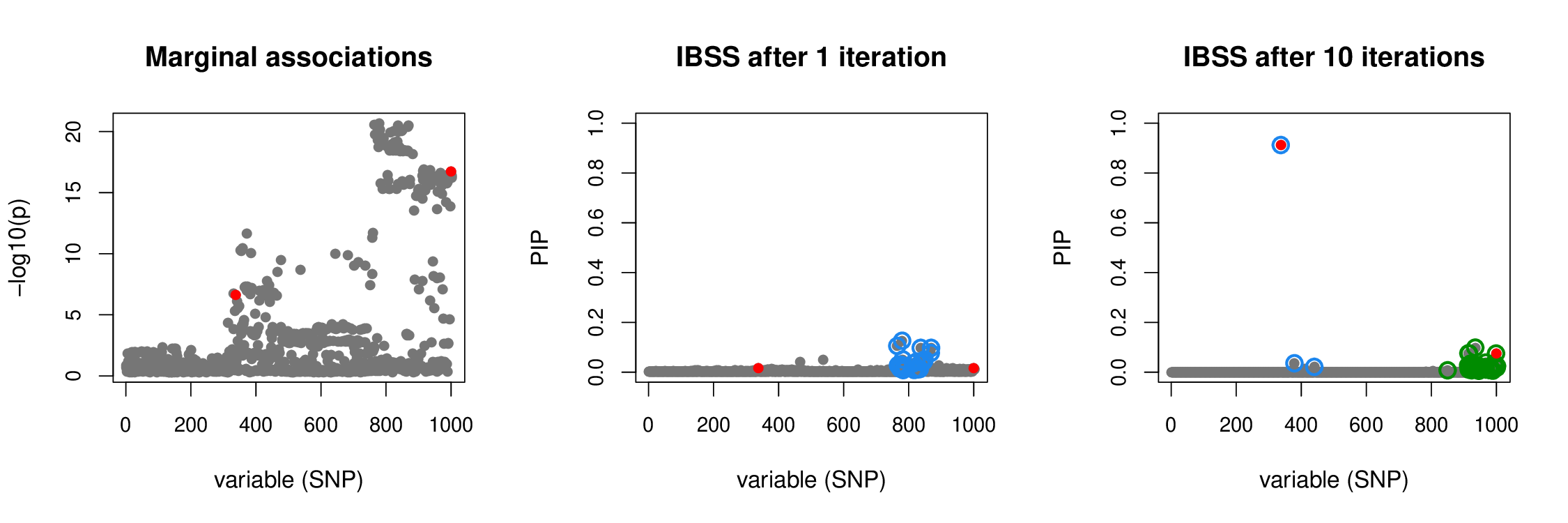For the middle panel, the CS looks like:

In :
print(fitted.one.iter$sets)  $cs
$cs$L1
 765 767 770 774 776 778 780 782 783 788 790 791 792 814 817 824 827 834 837
 838 847 849 868 869

$purity min.abs.corr mean.abs.corr median.abs.corr L1 0.779715 0.9254931 0.9270952$cs_index
 1

$coverage  0.95  The ultimately identified CS are: In : print(fitted$sets$cs)  $L2
  850  913  914  915  916  920  924  925  926  927  930  931  933  934  935
  942  946  948  951  952  962  967  968  979  980  982  983  985  988  989
  993  994  996  999 1000 1001 1002

$L3  337 379 440  L2 corresponds to the blue cluster around position 900. L3 corresponds to the cyan cluster around position 400. In both cases the simulated non-zero effect are captured by the confidence sets. The CS L2 has many variables. We check how correlated they are: In : fitted$sets$purity  A data.frame: 2 × 3 min.abs.corrmean.abs.corrmedian.abs.corr <dbl><dbl><dbl> L20.97223860.99397380.9949722 L30.85349810.91839930.8944609 The minimum absolute correlation is 0.97 for CS L2. So SuSiE behavior is reasonable. Notice that the strongest marginal association is at position In : which.max(abs(fitted$z))

780

LD pattern in the data-set can be visualized by:

In :
pdf('LDheatmap.pdf')
LDheatmap::LDheatmap(cor(dat$X[,100:200]),add.map=F,title="Correlation matrix\nfor variables 100 to 200") LDheatmap::LDheatmap(cor(dat$X[,350:450]),add.map=F,title="Correlation matrix\nfor variables 350 to 450")

In :
fitted$niter  23 In : susie_plot_iteration(fitted, 3, 'demo')  Creating GIF animation ... Iterplot saved to demo.gif  In : %preview demo.gif --width 80%  %preview demo.gif > demo.gif (205.8 KiB):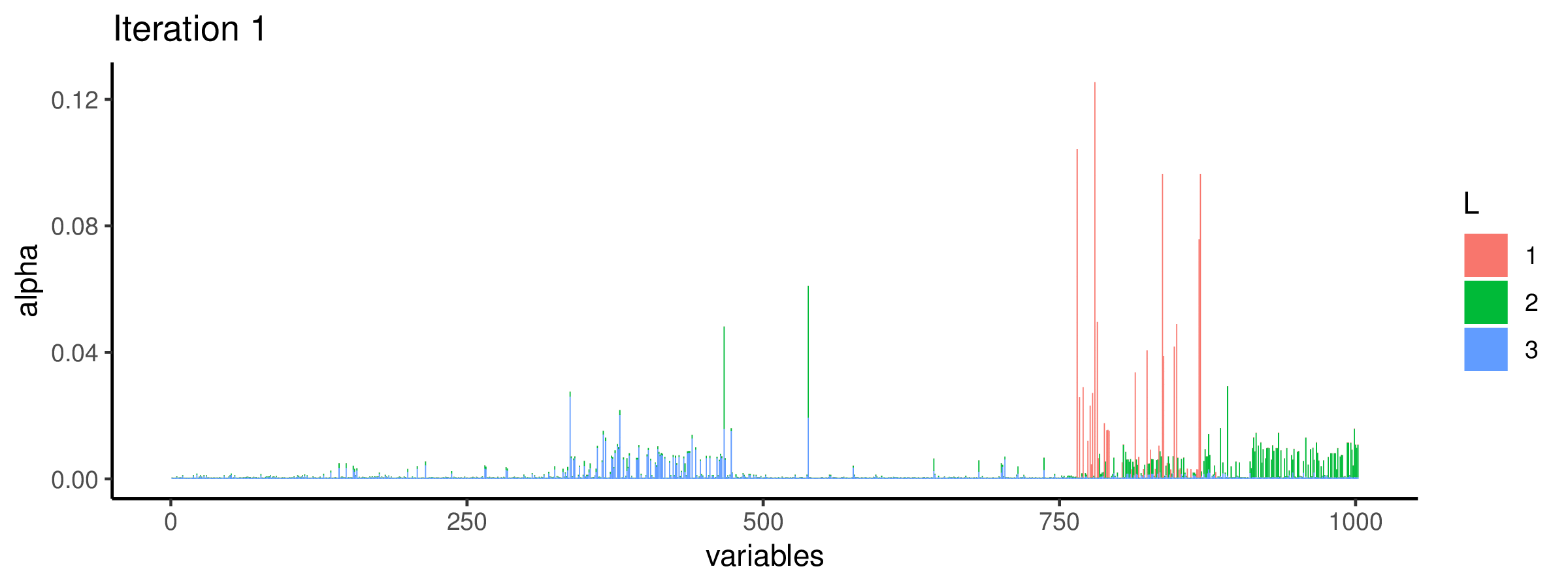At the first iteration, the variable with the top z-score was picked up by the first CS. But in later iterations, the first CS vanishes; the 2nd and 3rd CS correctly pick up the two "true" signals. ## SuSiE with L=2¶ We now set L=2 and see how SuSiE converges to the true signals, if capable: In : fitted = susie(dat$X, dat$Y[,r], L=2, estimate_residual_variance=TRUE, scaled_prior_variance=0.2, tol=1e-3, track_fit=TRUE, coverage = 0.95,min_abs_corr = 0.1) pdf('l2.pdf', width =5, height = 5, pointsize=16) susie_plot(fitted, y='PIP', b=b, max_cs=0.4, main = paste('SuSiE', length(fitted$sets$cs), 'CS identified')) dev.off()  png: 2 In : %preview l2.pdf -s png --dpi 150  %preview l2.pdf > l2.pdf (8.6 KiB):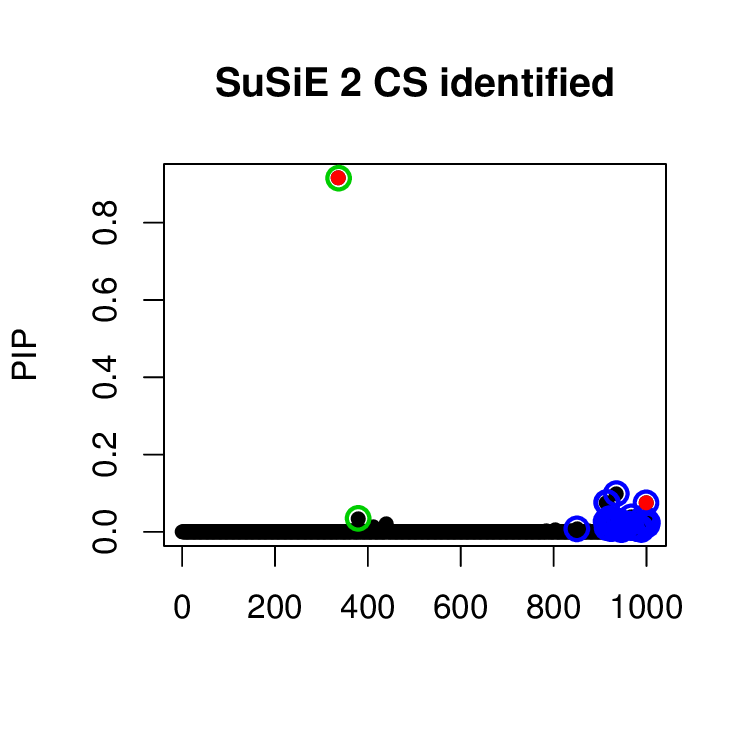In : fitted$niter

10
In :
susie_plot_iteration(fitted, 2, 'demo_l2')

Creating GIF animation ...
Iterplot saved to demo_l2.gif

In :
%preview demo_l2.gif --width 80%

%preview demo_l2.gif
> demo_l2.gif (150.8 KiB):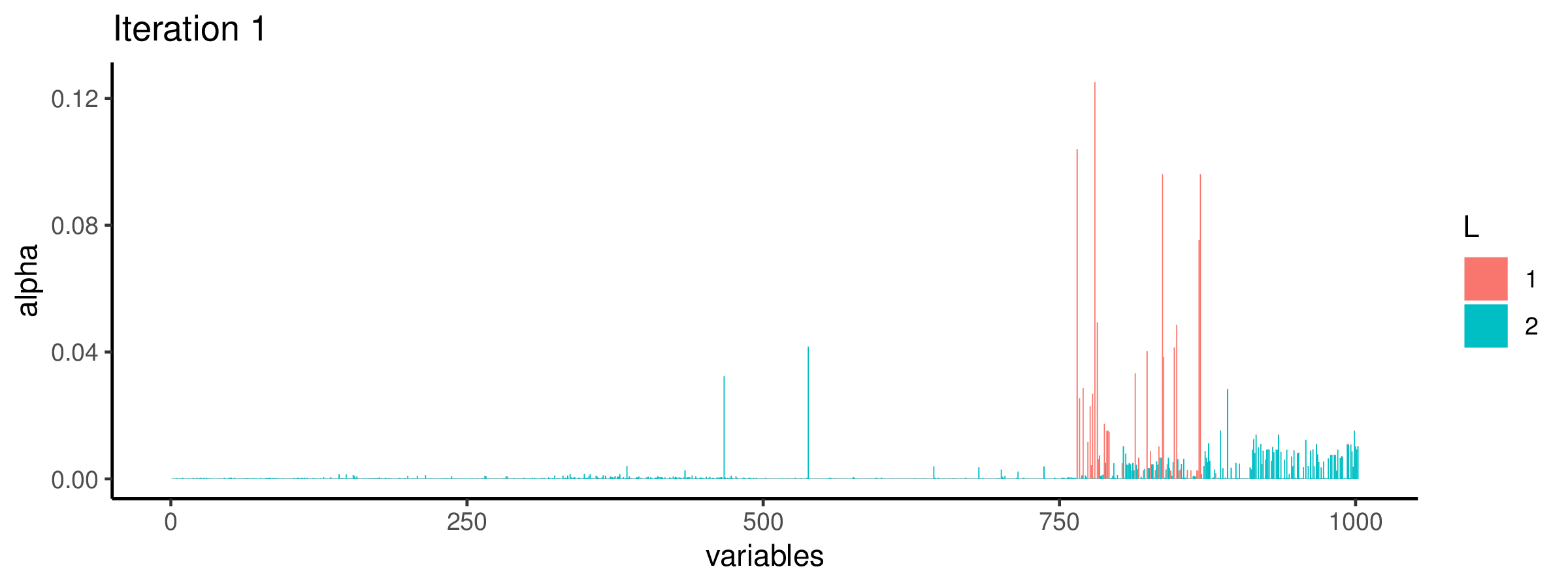It seems at the first iteration, the first CS picks up the induced wrong signal cluster, but both true signals were picked up by the 2nd CS which is quite wide. Later iterations removed the induced wrong cluster and the 2 CS properly converged to the two true signals as desired.

## Is the result robust to prior choice?¶

Here instead of fixing prior, I ask SuSiE to update prior variance

In :
fitted = susie(dat$X, dat$Y[,r], L=5,
estimate_residual_variance=TRUE,
estimate_prior_variance=TRUE,
tol=1e-3, track_fit=TRUE,
coverage = 0.95,
min_abs_corr = 0.1)

In :
pdf('est_prior.pdf', width =5, height = 5, pointsize=16)
susie_plot(fitted, y='PIP', b=b, max_cs=0.4, main = paste('SuSiE', length(fitted$sets$cs), 'CS identified'))
dev.off()

png: 2
In :
%preview est_prior.pdf -s png --dpi 150

%preview est_prior.pdf
> est_prior.pdf (8.6 KiB):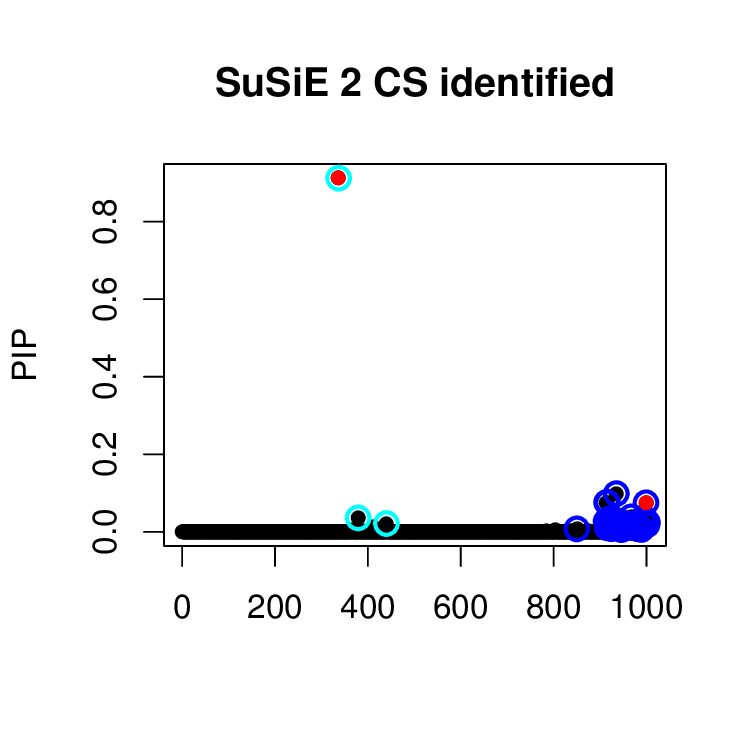Now I fix SuSiE prior to a smaller value (scaled prior is 0.005):

In :
fitted = susie(dat$X, dat$Y[,r], L=5,
scaled_prior_variance=0.005,
estimate_prior_variance=TRUE,
tol=1e-3, track_fit=TRUE,
coverage = 0.95,
min_abs_corr = 0.1)

In :
pdf('small_prior.pdf', width =5, height = 5, pointsize=16)
susie_plot(fitted, y='PIP', b=b, max_cs=0.4, main = paste('SuSiE', length(fitted$sets$cs), 'CS identified'))
dev.off()

png: 2
In :
%preview small_prior.pdf -s png --dpi 150

%preview small_prior.pdf
> small_prior.pdf (8.6 KiB):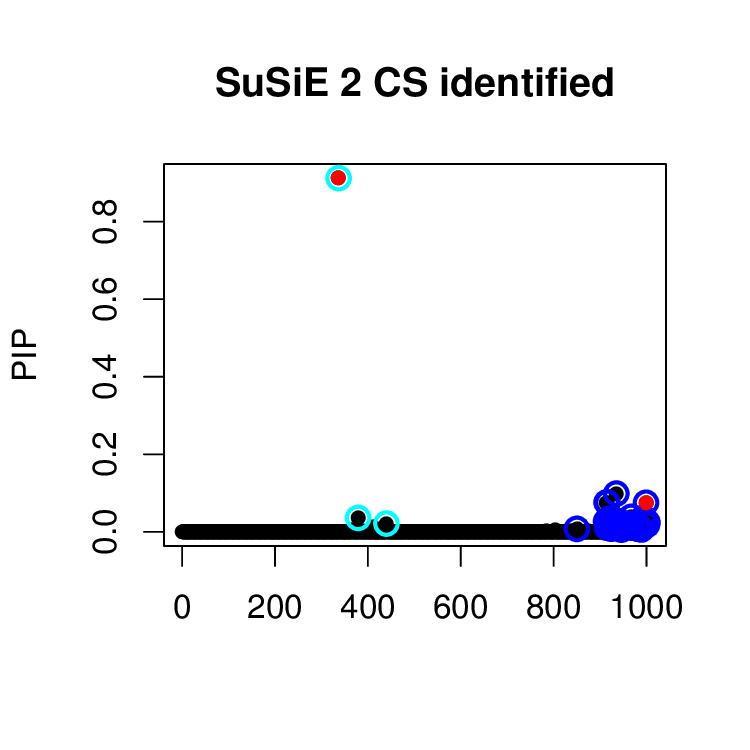Result remains largely the same.

## How other methods cope with this¶

In this folder we provide scripts to run CAVIAR, FINEMAP (version 1.1) and DAP-G on the same data-set for a comparison. We generate summary statistics and run these 3 other methods on the example.

In :
library(abind)
mm_regression = function(X, Y, Z=NULL) {
if (!is.null(Z)) {
Z = as.matrix(Z)
}
reg = lapply(seq_len(ncol(Y)), function (i) simplify2array(susieR:::univariate_regression(X, Y[,i], Z)))
reg = do.call(abind, c(reg, list(along=0)))
# return array: out[1,,] is betahat, out[2,,] is shat
return(aperm(reg, c(3,2,1)))
}
sumstats = mm_regression(as.matrix(dat$X), as.matrix(dat$Y))
saveRDS(list(data=dat, sumstats=sumstats), 'N2.with_sumstats.rds')

In :
export PATH=~/GIT/github/mvarbvs/dsc/modules/linux:$PATH Rscript ~/GIT/susieR/inst/code/finemap.R input=\"N2.with_sumstats.rds\" output=\"N2finemapping.FINEMAP\" args=\"--n-causal-max\ 2\" &> /dev/null  In : export PATH=~/GIT/github/mvarbvs/dsc/modules/linux:$PATH
Rscript ~/GIT/susieR/inst/code/caviar.R input=\"N2.with_sumstats.rds\" output=\"N2finemapping.CAVIAR\" args=\"-c\ 2\ -g\ 0.01\" &> /dev/null

In :
export PATH=~/GIT/github/mvarbvs/dsc/modules/linux:$PATH python ~/GIT/susieR/inst/code/dap-g.py N2.with_sumstats.rds N2finemapping.DAP -ld_control 0.20 --all &> /dev/null  Now we look at these results. In : caviar = readRDS("N2finemapping.CAVIAR.rds") finemap = readRDS("N2finemapping.FINEMAP.rds") dap = readRDS("N2finemapping.DAP.rds")  ### CAVIAR results¶ #### PIP¶ In : snp = caviar[]$snp
pip = snp[order(as.numeric(snp$snp)),]$snp_prob
pdf('caviar_fm.pdf', width =5, height = 5, pointsize=16)
susie_plot(pip, y='PIP', b=b, main = 'CAVIAR')
dev.off()

png: 2
In :
%preview caviar_fm.pdf -s png --dpi 150

%preview caviar_fm.pdf
> caviar_fm.pdf (8.9 KiB):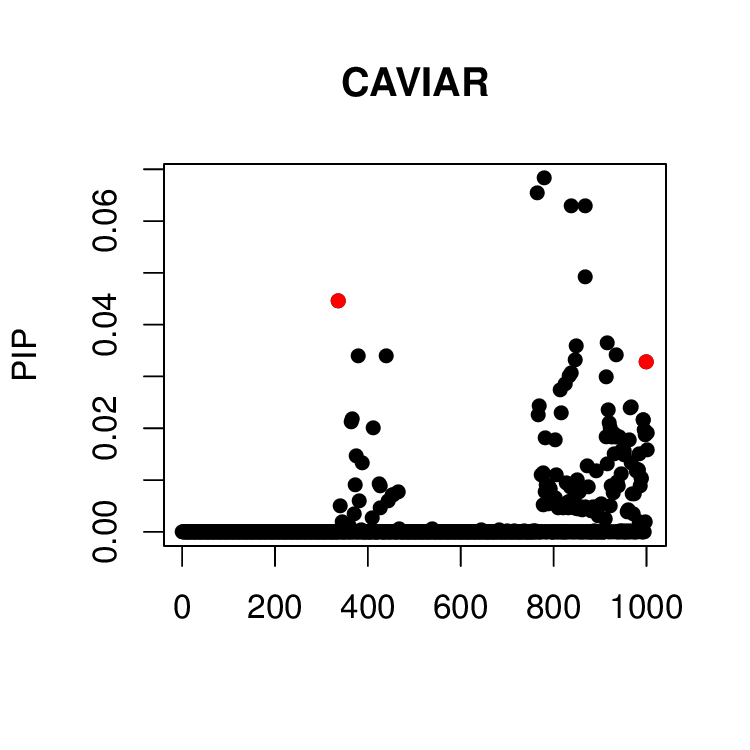#### 95% CS¶

CAVIAR provides single 95% confidence sets as follows:

In :
print(caviar[]$set)    "780" "765" "837" "869" "868" "337" "916" "849" "935" "379"  "440" "847" "999" "838" "834" "914" "824" "814" "770" "967"  "964" "918" "817" "767" "367" "993" "994" "365" "920" "922"  "411" "996" "1000" "1002" "930" "997" "926" "913" "925" "927"  "933" "934" "942" "782" "962" "804" "948" "1001" "952" "985"  "931" "951" "375" "968" "387" "915" "873" "979" "980" "982"  "983" "892" "977" "778" "946" "806" "774" "776" "988" "989"  "850" "937" "827" "424" "372" "784" "426" "924" "940" "788"  "987" "836" "842" "874" "791" "790" "792" "783" "855" "465"  "929" "969" "973" "452" "455" "803" "841" "382" "443" "832"  "789" "809" "812" "902" "777" "899" "921" "341"  In : any(which(b>0) %in% caviar[]$set)

TRUE

It is a wide CS, although it does capture the causal SNPs.

#### Choice of prior¶

When I set CAVIAR non-centrality parameter to 10 instead of the default 5.2, I do get an improved result,

In :
%preview /tmp/CAVIAR.ncp.hack.png

%preview /tmp/CAVIAR.ncp.hack.png
> /tmp/CAVIAR.ncp.hack.png (30.4 KiB):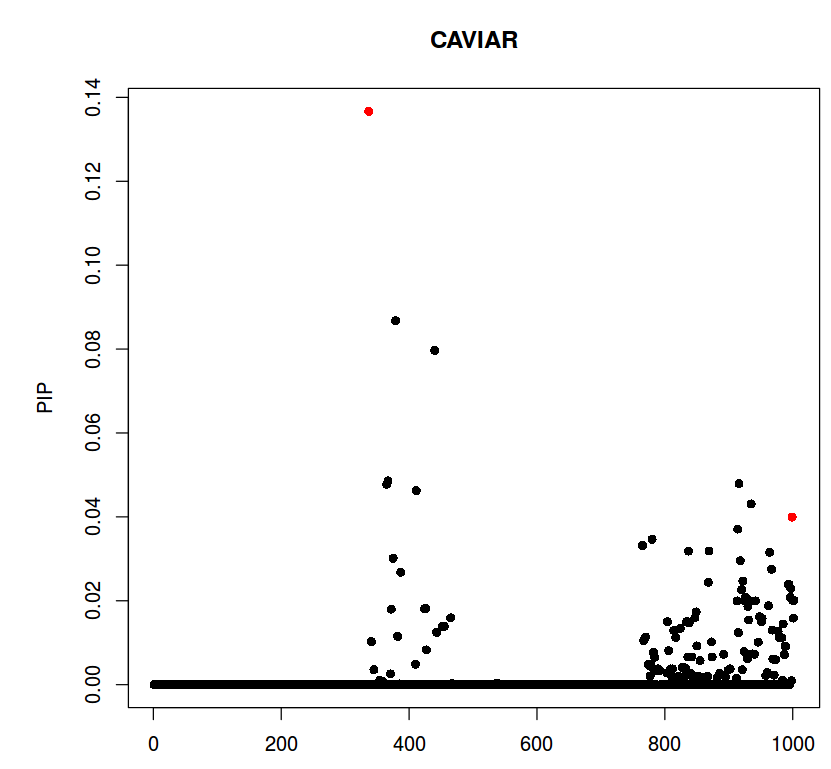Analysis procedure not shown because CAVIAR by default does not allow configuration of NCP (need to change the code and re-compile the binary).

### FINEMAP results¶

In :
snp = finemap[]$snp pip = snp[order(as.numeric(snp$snp)),]$snp_prob pdf('finemap_fm.pdf', width =5, height = 5, pointsize=16) susie_plot(pip, y='PIP', b=b, main = 'FINEMAP') dev.off()  png: 2 In : %preview finemap_fm.pdf -s png --dpi 150  %preview finemap_fm.pdf > finemap_fm.pdf (8.5 KiB):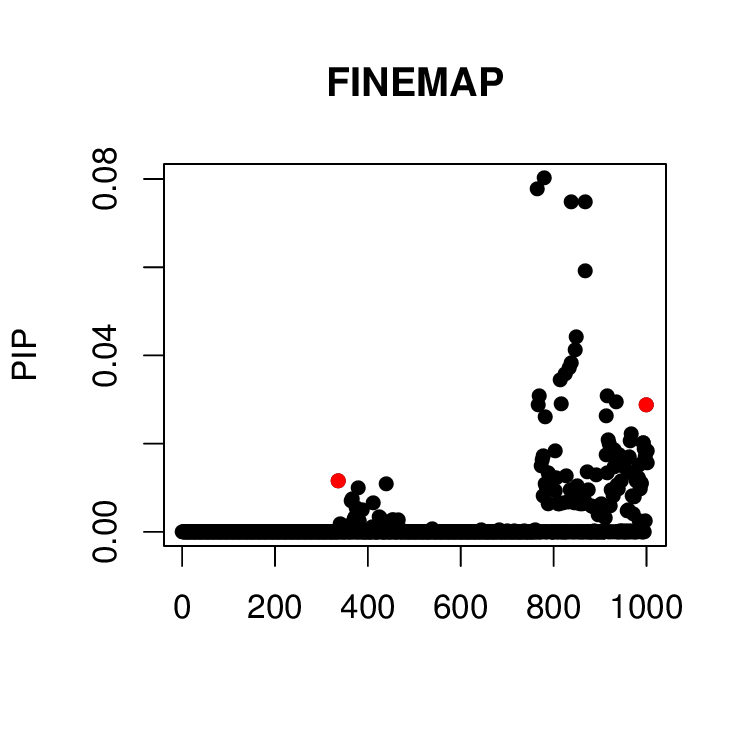#### 95% CS¶ For each configuration, FINEMAP provides the corresponding configuration probability: In : head(finemap[]$set, 10)

rankconfigconfig_probconfig_log10bf
1 780,916 0.00243037615.22015
2 780,935 0.00233733715.20320
3 780,999 0.00233203015.20221
4 765,999 0.00220979515.17883
5 765,935 0.00220849815.17858
6 765,916 0.00217186115.17131
7 869,999 0.00213420115.16371
8 837,999 0.00213420115.16371
9 869,935 0.00213331815.16353
10 837,935 0.00213331815.16353

It requires post-processing to come up with a single 95% set similar to that of CAVIAR with all 3 causal variables captured. The result from running finemap.R provided has been truncated to the minimum set of configurations having cumulative probability of 95%. But the top 10 configurations completely missed the causal signals.

#### Choice of prior¶

The default prior for FINEMAP is 0.05 (for the Gaussian standard deviation). Knowing from simulation parameters of the true effect size, we increase it to 0.1.

In :
export PATH=~/GIT/github/mvarbvs/dsc/modules/linux:$PATH Rscript ~/GIT/susieR/inst/code/finemap.R input=\"N2.with_sumstats.rds\" output=\"N2finemapping.FINEMAP.p1std\" args=\"--n-causal-max\ 2\ --prior-std\ 0.1\" &> /dev/null  In : finemap = readRDS("N2finemapping.FINEMAP.p1std.rds") snp = finemap[]$snp
pip = snp[order(as.numeric(snp$snp)),]$snp_prob
pdf('finemap_bigger_prior.pdf', width =5, height = 5, pointsize=16)
susie_plot(pip, y='PIP', b=b, main = 'FINEMAP')
dev.off()

png: 2
In :
%preview finemap_bigger_prior.pdf -s png --dpi 150

%preview finemap_bigger_prior.pdf
> finemap_bigger_prior.pdf (8.3 KiB):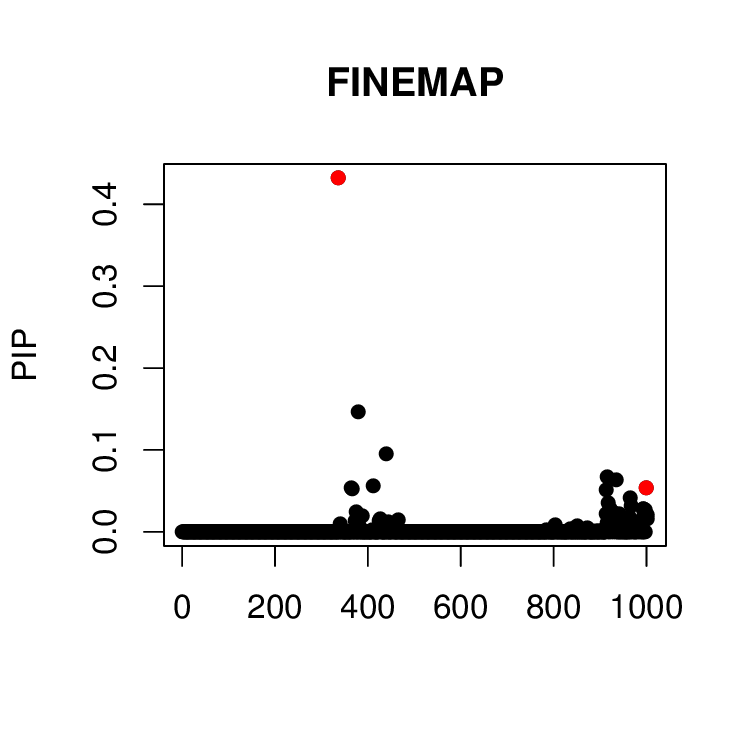In :
head(finemap[]$set, 10)  rankconfigconfig_probconfig_log10bf 1 337,935 0.0334211623.07379 2 337,916 0.0289438623.01132 3 337,914 0.0270004922.98114 4 337,999 0.0260478622.96554 5 337,967 0.0165378522.76824 6 337,993 0.0141845022.70158 7 337,994 0.0141805722.70146 8 337,920 0.0135378622.68131 9 379,964 0.0135253422.68091 10 337,926 0.0122543822.63806 The reported best configuration is closer to the true effects. ### DAP-G results¶ #### PIP¶ In : snp = dap[]$snp
pip = snp[order(as.numeric(snp$snp)),]$snp_prob
pdf('dap_fm.pdf', width =5, height = 5, pointsize=16)
susie_plot(pip, y='PIP', b=b, main = 'DAP-G')
dev.off()

png: 2
In :
%preview dap_fm.pdf -s png --dpi 150

%preview dap_fm.pdf
> dap_fm.pdf (8.2 KiB):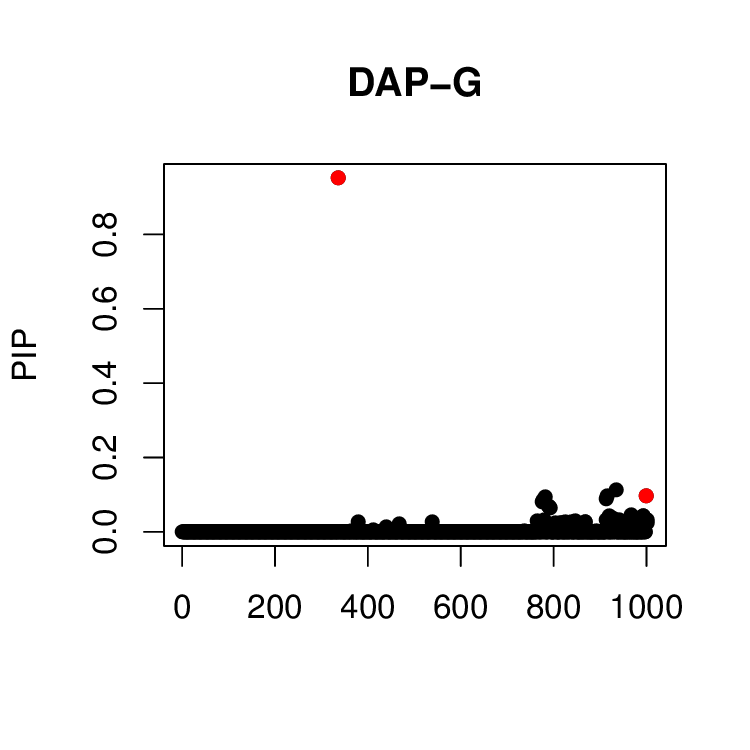The default prior works

#### 95% CS¶

Similar to SuSiE, DAP-G provides per-signal 95% CS:

In :
dap[]$set  clustercluster_probcluster_avg_r2snp 01 1.000000 0.855 782,778,776,788,790,791,792,780,765,847,869,837,868,824,849,817,838,834,770,814,767,803,783,774,827 12 1.000000 0.966 935,916,999,914,967,993,994,920,926,925,913,927,933,934,942,996,1002,1000,962,1001,930,985,804,892 23 1.000000 0.821 337,379,440,411,365,367 34 0.055970 0.558 538,467,947,737,965,911,956 45 0.000072 1.000 157 Using an average$r^2$filter of 0.2 ($r=0.44$, compared to SuSiE's$r=0.1\$ in earlier analysis), it reports five 95% CS; the first 3 CS contain causal signals. Also the first CS is wider.

© 2017-2018 authored by Gao Wang at Stephens Lab, The University of Chicago

Exported from manuscript_results/pedagogical_example.ipynb committed by Gao Wang on Wed Feb 12 10:59:05 2020 revision 22, 036919f